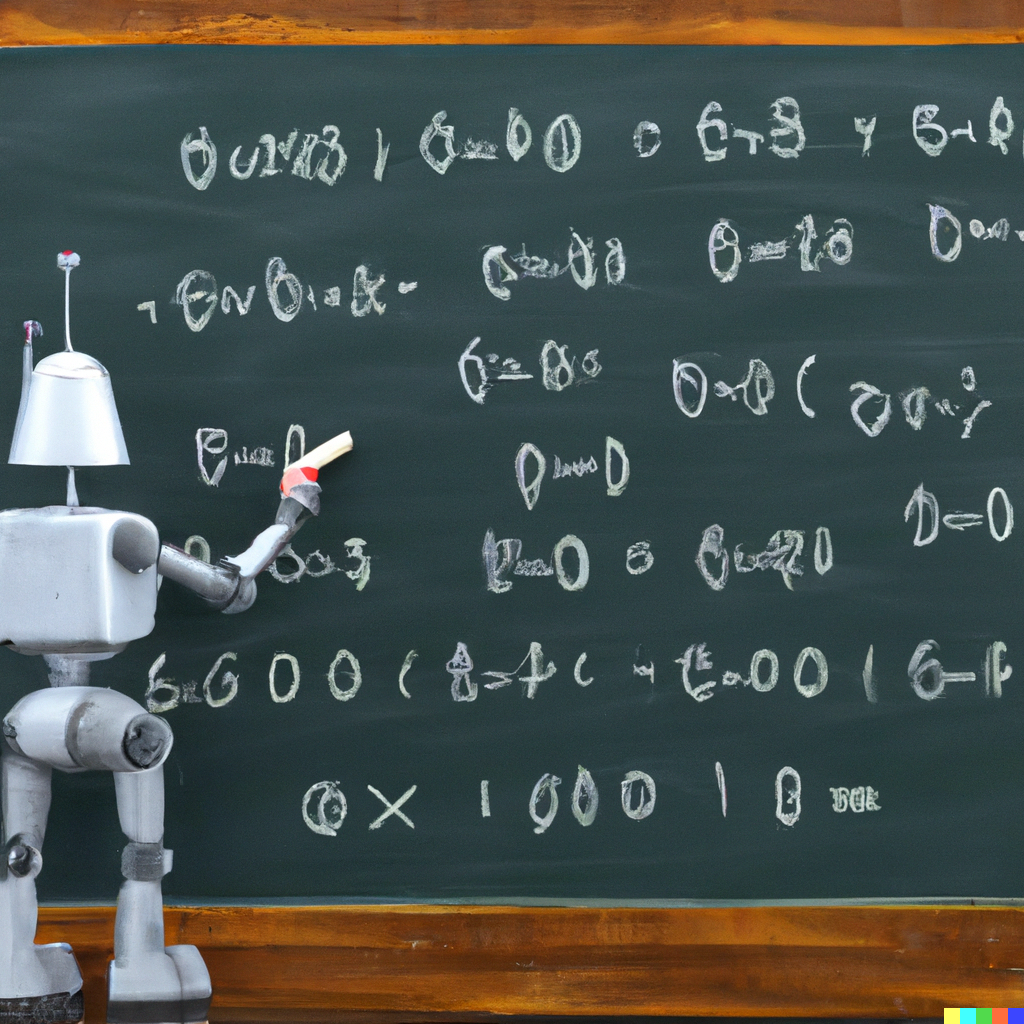## Matching Mathematical Statements to their Proofs

### Description

We develop a model to match mathematical statements to their corresponding proofs. This task can be used in areas such as mathematical information retrieval. Our work comes with a dataset for this task, including a large number of statement-proof pairs in different areas of mathematics.### Paper

```@inproceedings{li-23,
author = "W. Li and Y. Ziser and M. Coavoux and S. B. Cohen",
title = "BERT is not The Count: Learning to Match Mathematical Statements with Proofs",
booktitle = "Proceedings of {EACL}",
year = "2023"
}
```